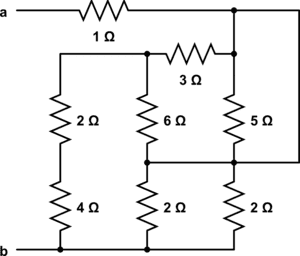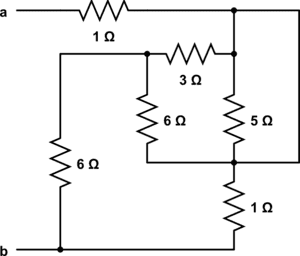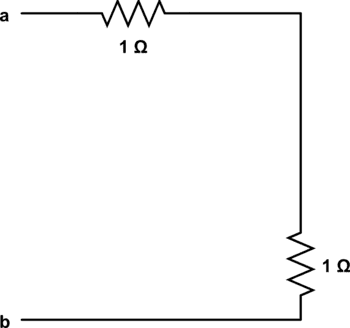# Equivalent Resistance - Resistors Intertwined

• Hurricane9
In summary, the problem the student was having was that they could not figure out how to simplify the circuit. The 3 ohm resistor was shorted and the remaining resistors were not in parallel or series.f

## Homework Statement

Find the equivalent resistance between terminals a and b## Homework Equations

Series: Req = summation of resistors

Parallel 1/Req = summation of 1/resistances

## The Attempt at a Solution

So I figured out the easy ones, and so I'm here at the moment:And that is where I am stuck. The problem I am finding is that everything is intertwined and I can't figure out how to simplify anymore.

For example, I feel the next step is to combine the 6 ohm and the 5 ohm resistor in the middle. But I can't say they are in parallel, with the 3 ohm and the 6 ohm being in series, as the other 6 ohm resistor is there tacked on. So the 3 ohm resistor is in parallel with the two 6 ohm ones. But again, I can't use the parallel equal for those because the right 6 ohm resistor is connected to the 1 ohm resistor, and I can't say the 6 ohm and the 1 ohm are in series, because the 5 ohm and the top 1 ohm are also connected to it. And I can't say the top 1 ohm, the 3+6+6 ohm portion, and the 5 ohm portion are all in parallel, because the 1 ohm connects at the top and at the bottom.

Basically, I have no clue where to start. I've been looking up example problems on the internet all afternoon and none of them I've found have had these issues.

Thanks in advance for the help! I am sure I can figure it out if someone could push me in the right direction. I am just not sure where my understanding of the concepts is wrong.

Your next step is to redraw the diagram - simplifying it.
i.e. there is an unneeded node between the 1, 3, and 5 ohm resistors.
Notice that the loop around the 5ohm resistor is a short - it basically removes a (bunch of?) resistor(s) from the circuit - totally changing how it looks.

I suspected the trick was with that, but the idea of a short hasn't really been covered enough for me to be sure that was the case.

Does it mean I can essentially take everything out but two 1 ohm resistors?Or is that too much taken out? I am unsure whether that 3 ohm resistor is shorted. The 5 definitely is, right?

Last edited:
Here's a hint: look at the 6 ohm and 3 ohm resistor on the right side.

Last edited:
I'm not sure what you mean.

I'm still unsure about the short. A resistor has to be in parallel with the short to be shorted, right? So I don't believe the 3 ohm and the two 6 ohm resistors are shorted. If this is true, then I get to a point where the remaining resistors are not in parallel or series, so I can't proceed. So I can't see how I can solve this problem without the 3 being shorted, but I don't understand why it would be.

Since the 5 ohm resistor can be removed (it's shorted), the 3 ohm and the 6 ohm resistors are in ______? (try redrawing the circuit with the 5 ohm resistor removed)

Last edited:
•1 person
That's right - I like to redraw circuits with one terminal at the top and the other at the bottom, then the circuit makes a kind-of flow diagram from top to bottom.

Don't be frightened to redraw the circuits several times ... I use a large window and a whiteboard marker.

AH, they are in parallel.

Great, I can solve it now! Thanks a lot!